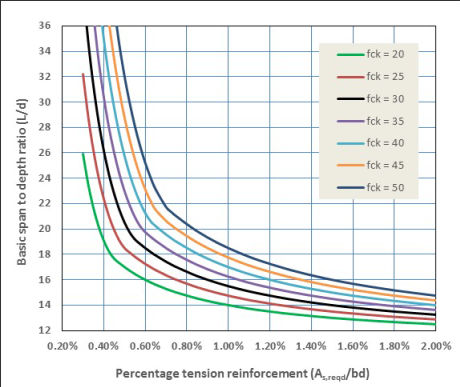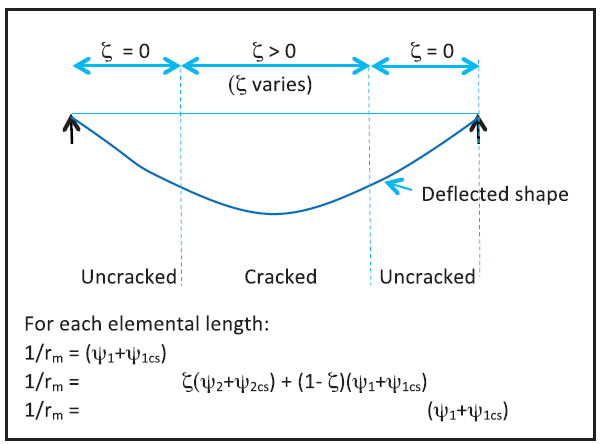# Deflection

Eurocode 2 has two alternative methods for checking deflection. Either:

• a limiting span-to-depth ratio may be used or
• the theoretical deflection can be assessed using the expressions given in the Code

The span-to-effective-depth (L/d) method

In simple terms, the current EN1992 L/d method means verifying that:

 Allowable L/d = N x K x F1 x F2 x F3 ≥ actual L/d

where

 N = basic span-to-effective-depth ratio derived for K = 1.0 and p'. from the formulae : if ρ0 ≤ ρ N = L/d = K[11 + 1.5fck0.5 ρ 0/ ρ + 3.2 fck0.5(ρ 0/ ρ – 1)1.5] or if ρ0 > ρ N = L/d = K[11 + 1.5 fck 0.5 ρ0/( ρ – ρ') + fck0.5(ρ'/ ρ0)0.5/12] For ρ’ =0, N may be determined from Figure 1 where L = span d = effective depth ρ0 = fck0.5/1000 ρ  = As,req/bd ρ’ = As2/bd K = factor to account for structural system. See Table 1 F1 = factor to account for flanged sections. When beff/bw = 1.0, factor F1 = 1.0. When beff/bw is greater than 3.0, factor F1 = 0.80. For values of beff/bw between 1.0 and 3.0, interpolation may be used. . F2 = factor to account for brittle partitions in association with long spans. Generally F2 = 1.0 but if brittle partitions are liable to be damaged by excessive deflection, F2 should be determined as follows: a) in flat slabs in which the longer span is greater than 8.5 m, F2 = 8.5/leff b) in beams and other slabs with spans in excess of 7.0 m, F2 = 7.0/leff F3 = factor to account for service stress in tensile reinforcement = 310/ss1,3a. It is considered conservative to assume that 310/ss = 500 As,prov/(fykAs,req)  where ss  = tensile stress in reinforcement at mid-span (at support for cantilevers) under design load at SLS. Notes In MC2010 this expression is  given in Expression (7.6-23) as 250/ss = 500 As,prov/(fykAs,req) Factors F1, F2 and F3 have been used here for convenience, they are not symbols used in EN1992-1-1. According to the Notes to Table NA.5 of the UK NA, the ratio, F3, is restricted to ≤ 1.5 where ss  is calculated using the characteristic value of serviceability load. Warnings are given that the values of K may not be appropriate when formwork is struck at an early age. L/d may not exceed 40K.

Figure 7 Basic span-to-effective-depth ratios, N, for K = 1, ρ' = 0Extract from How to Design Concrete Structures using Eurocode 2 (page 27, Figure 5)

 Table 1 K factors to be applied to basic ratios of span to effective depth for structural system Element K Simply supported beams or slabs 1.0 End span of continuous beams or slabs 1.3 Interior spans of continuous beams or slabs 1.5 Flat slabs (based on longer span) 1.2 Cantilevers 0.4

The use of L/d method ‘will be adequate for avoiding deflection problems in normal circumstances’

## The rigorous method of assessing deflection

A section will crack if it experiences a serviceability moment exceeding its moment capacity at the time, Mcr(t). If a section is cracked, then its inertia is much less than that of the uncracked section and so curvature is much greater in cracked sections. Cracked sections and greater degrees of cracking lead to larger curvatures and deflections. See Figure 3.

Figure 3 Typical moment - curvature responseEconomically designed horizontal elements act somewhere between wholly uncracked and wholly cracked. Slabs tend to be less highly stressed and are cracked along only part of their spans. Beams tend to be highly stressed and cracked along much of their spans. Actions are applied at different times and these actions may or not cause cracking depending upon the flexural tensile strength of the concrete at the time. Once cracked, a section is assumed to stay cracked but some tensile stiffening occurs in the concrete between cracks. So the mean inertia of the segment is somewhere between those for wholly un-cracked or fully cracked sections. When considering curvatures, these different actions incur different creep coefficients, which affect the applicable effective modulus of the concrete used in assessing curvatures.

EN 1992-1-1 (and MC2010) states that an adequate prediction of behaviour and the mean curvature in a discreet element is given by

 1/rm = ζ(ψ2+ψ2cs) + (1- ζ)(ψ1+ψ1cs) where rm = mean radius z = 1- b(Mcr/M)2 where b = 1.0 for short –term and 0.5 for long-term loading. For construction loads, conservatively b = 0.70 Mcr = cracking moment M = Moment y1 = M/EceffI1 = curvature of uncracked section y2 = M/EceffI2 = curvature of cracked section where Eceff = Ecm/(1+ f) where Ecm = modulus at 28 days f  = creep coefficient I1, I2 = inertias y1cs, y2cs = shrinkage curvatures

Curvature in a simply supported slabThis ‘rigorous’ method is described in greater detail elsewhere e.g. Concrete Society.  Deflections in concrete slabs and beams, (2005) TR 58, or How to design concrete structures using Eurocode 2: Deflection Calculations. It is backed by site based research.

Greater accuracy may be achieved by considering small increments of span and computing relevant curvatures and thus overall deflections. The method involves numerical integration, which is tedious by hand but can, of course, be undertaken by computer, notably by spreadsheet software.

Sep

12:30 - 13:30

Free

Sep

18:00 - 20:00

Free

Sep

09:30 - 16:00

Free